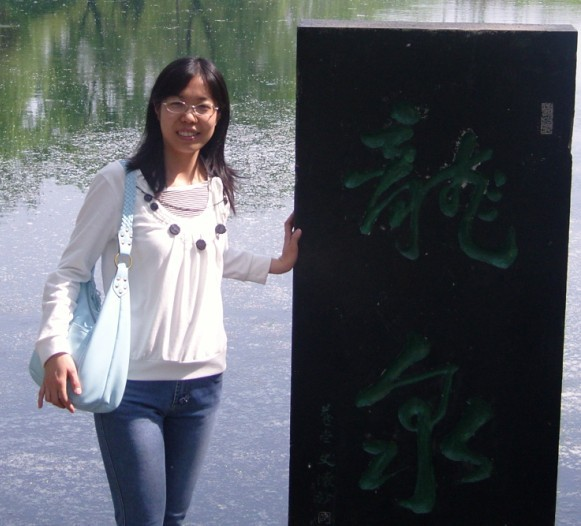﻿ Qingfeng Sun-Number Theory in Shandong UniversityQingfeng Sun

Curriculum Vitae

### Family name and given name

Family name: Sun;
given name:QingfengSchool of Mathematics  and Statistics
Shandong University at  Weihai
Weihai, Shandong  264209
China
Email: qfsun(at)sdu.edu.cn

### Education

Ph.D.: Shandong University, 2010
B.S.: Shandong University, 2005

### Research Interests

Exponential sums, automorphic L-functions

### Publications

 On determination of GL_3 cusp forms，to appear in Acta Arith.
 Non-vanishing of derivatives of GL(3) \times GL(2) L-functions, to appear in
Proc. Amer. Math. Soc.
 Cancelation of cusp forms coefficients over Beatty sequences on  GL(n),
to appear in Can. Math. Bull.
 Selberg's Integral for Ramanujan Automorphic Representation, to appear in      Acta Math. Sin. (Engl. Ser.)
 Nonlinear exponential twists of the  Liouville function, Cent. Eur. J. Math. 9 (2)      (2011), 328-337.
 On cusp form coefficients in nonlinear exponential sums, Quart. J. Math.
61 (3) (2010), 363-372.
 (with Q.H. Pi) Oscillations of cusp form coefficients in  exponential sums,
Ramanujan J. 21 (2010), 53-64.
 (with Q.H. Pi) Oscillations of cusp form  coefficients over primes, Acta. Appl.      Math. 109 (2010), 1155-1163.
 (with H.C. Tang) Hua's theorem with s almost equal prime variables, Acta
Math. Sin. (Engl. Ser.) 25 (7) (2009),1145-1156.

[ back ]